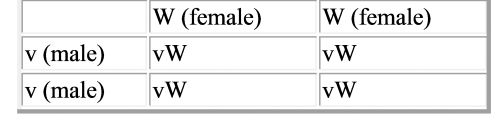# Working through analysis of 1st cross in Virtual Fly Lab

At the end of the Virtual Fly lab, it tells you to do a chi-square with your data to see if your crosses fit the null hypothesis.

For example, your first cross is vestigial wings (male) crossed with wildtype (female). You did a F1 and then a F2 cross and get the following data:

F1:
– – 607 males and 600 females, all wildtype

F2:
– – 494 males and 458 females, all wild type
– – 146 males and 150 females, vestigial wings

From looking at this, you can deduce that the vestigial gene is recessive, and is not sex-linked. Your null hypothesis is that any difference between your data and what you would expect from a classic autosomal recessive gene is due to chance and nothing is acting on the populations to alter the actual outcome.

Okay, let’s do a quick Punnet Square to get our expected data.
We will use v = vestigial and W = wildtypeAs you can see, all the progeny of the first cross will be of genotype vW and of phenotypr wildtype. Remember, we are assuming that the vestigial wings are autosomal recessive here (from looking at our rough data) but it is still a guess.

Anyway, the F2 cross would ostensibly be vW x vW, which will be the following square:So, we would expect 25% of our progeny to have vestigial wings (the vv genotype), with 75% having the wildtype phenotype (but a mixed genotype since we cannot tell the difference between vW and WW).

– – 494 males and 458 females, all wild type (total 952)
– – 146 males and 150 females, vestigial wings (total 296)

We don’t need to keep the males and females separate anymore, so we can use the totals. Our total number of flies = 1248
If we had a perfect cross, we would have  25% of the total number of flies = expected # of homozygous vv
And 75% of the total number of flies = wildtype phenotype

So, our expected data would be:
25% of 1248 = 312 of the vestigial phenotype
75% of 1248 = 936 of wildtype phenotype

Our equation is:
X2(chi-square)  = the sum of ￼
where:
o = observed number of individuals
e = expected number of individuals

Our chi-square value = (952 – 936)(952-936)/936 + (296-312)(296-312)/312
Chi-square = 1.094
Degrees of freedom: we have 2 categories (vestigial and wildtype) so our DF = 1
Looking at the tables, our p=0.05 = 3,84
Since our value is less than the p=0.05 value, that means we can assume that the difference between our data and the expected data is due to chance, and that our null hypothesis is verified. Or in other words, our cross fits the autosomal recessive pattern.